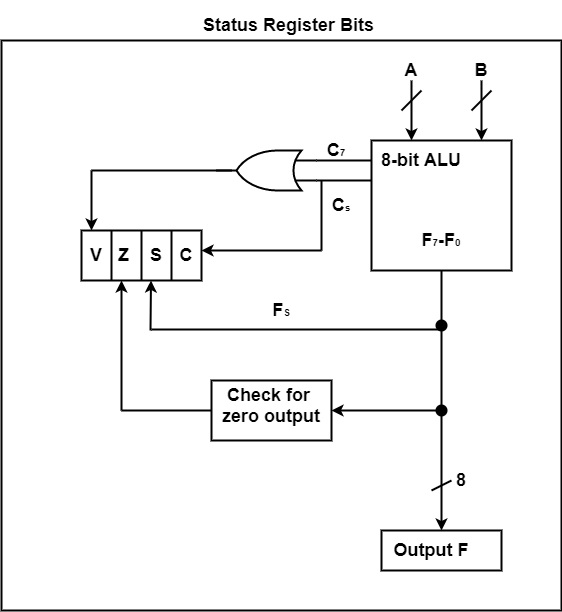# What are the conditions of Status Bits in Computer Architecture?

The status register comprises the status bits. The bits of the status register are modified according to the operations performed in the ALU. The figure displays a block diagram of an 8-bit ALU with a 4-bit status register.If the end carry C8 is 1, then carry (C) is set to 1. If C8 is 0, then C is cleared to 0.

If the highest order bit F7 is 1, then Sign (S) is set to 1. If F7 is 0, then S is set to 0.

If the output of ALU is 0, then zero (Z) is set to 1, otherwise, Z is set to 0.

If the XOR of the last two carries is equal to 1, then overflow (V) is set to 1, otherwise, V is cleared to 0.

The result of the 8-bit ALU operation is either 127 or -127.

Z is a status bit used to indicate the result obtained after comparing A and B. Here, the XOR operation is used to compare two numbers (Z = 0 if A = B).

## Conditional Branch Instruction

The conditional branch instruction checks the conditions for branching using the status bits. Some of the commonly used conditional branch instructions are shown in the table.

Conditional Instructions

MnemonicConditionTested Condition
BZBranch if zeroZ = 1
BNZBranch if not zeroZ = 0
BCBranch if carryC = 1
BNCBranch if no carryC = 0
Branch if zeroBranch if plusS = 0
BMBranch if minusS = 1
BVBranch if overflowV = 1
BNVBranch if no overflowV = 0
Unsigned compare conditions (A –B)

BHIBranch if higherA > B
BHEBranch if higher or equalA ≥ B
BLOBranch if lowerA < B
BLOEBranch if lower or equalA ≤ B
BEBranch if equalA = B
BENBranch if not equalA / B
Signed compare conditions (A – B)

BGTBranch if greater thanA > B
BGEBranch if greater or equalA ≥ B
BLTBranch if less thanA < B
BLEBranch if less or equalA ≤ B
BEBranch if equalA = B
BENBranch if not equalA / B

Thus, when the status condition is true, the program control is transferred to the address specified in the instruction, otherwise, the control continues with the instructions that are in the subsequent locations. The conditional instructions are also associated with the program control instructions such as jump, call, or return.

The zero status bit checks if the result of the ALU is zero or not. The carry bit checks if the most significant bit position of the ALU has a carryout. It is also used with rotate instruction to check whether or not the bit is shifted from the end position of a register into a carry position.

The sign bit indicates the state of the most significant bit of the output from the ALU (S = 0 denotes positive sign and S = 1 denotes negative sign). The branch if plus and branch if minus is used to check whether the value of the most significant bit represents a sign or not. The overflow and underflow instructions are used in conjunction with arithmetic operations performed on signed numbers.

The higher and lower words are used to denote the relations between unsigned numbers, whereas the greater and lesser words are used to denote the relations between signed numbers.